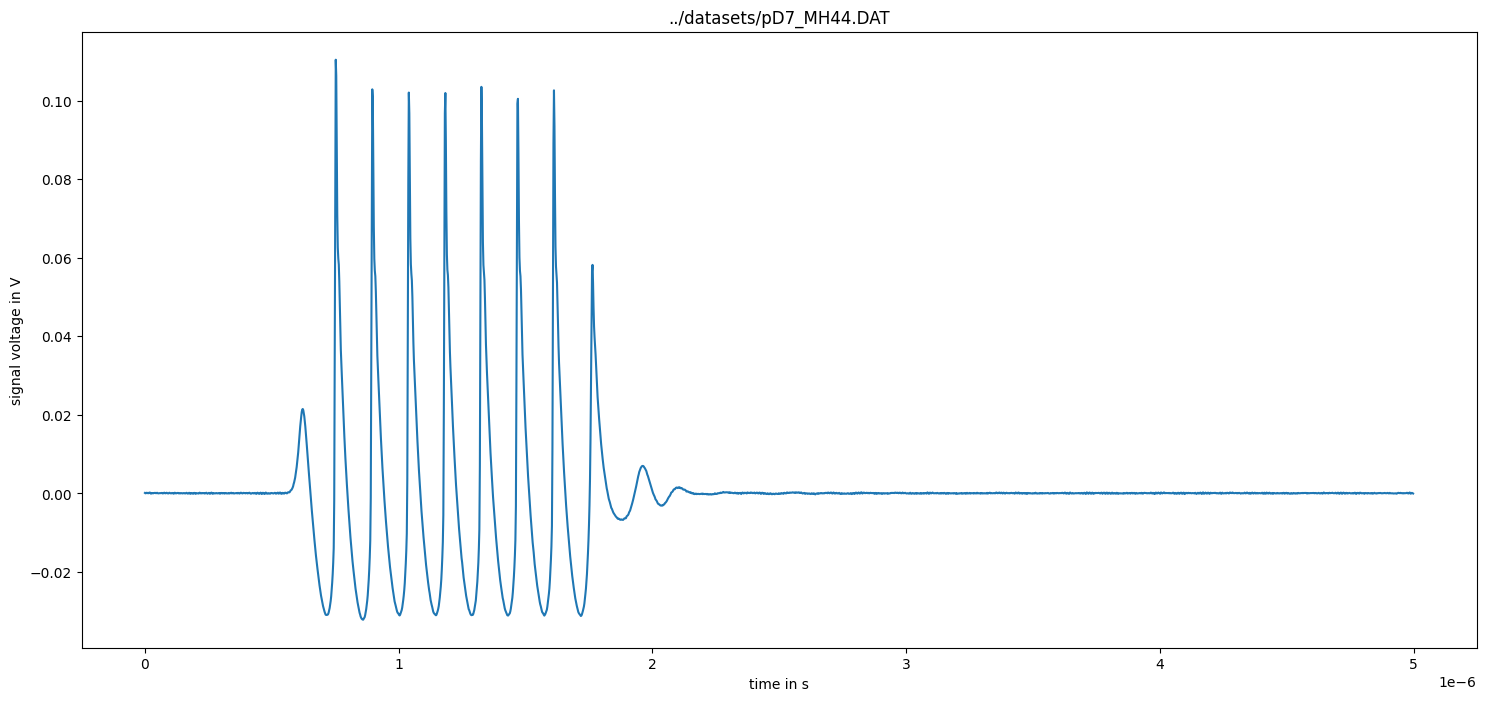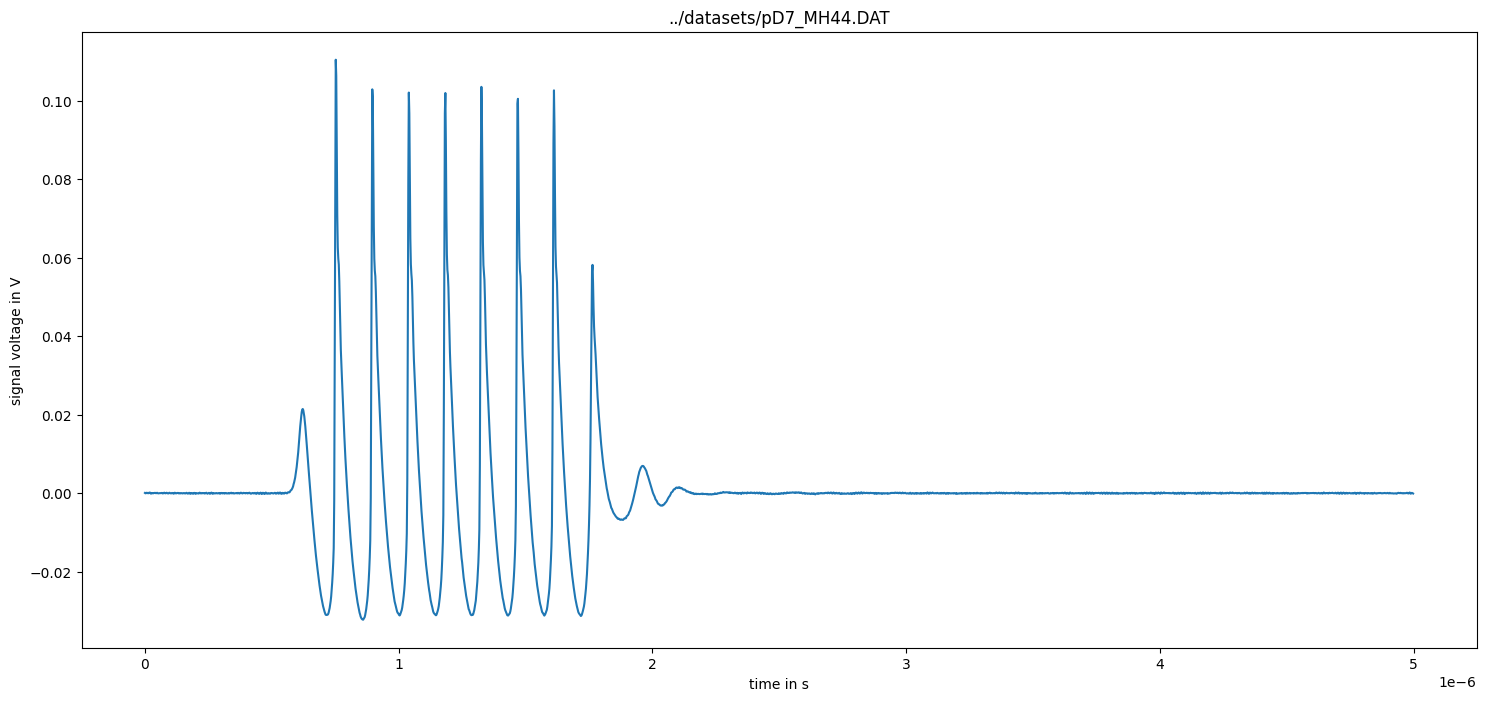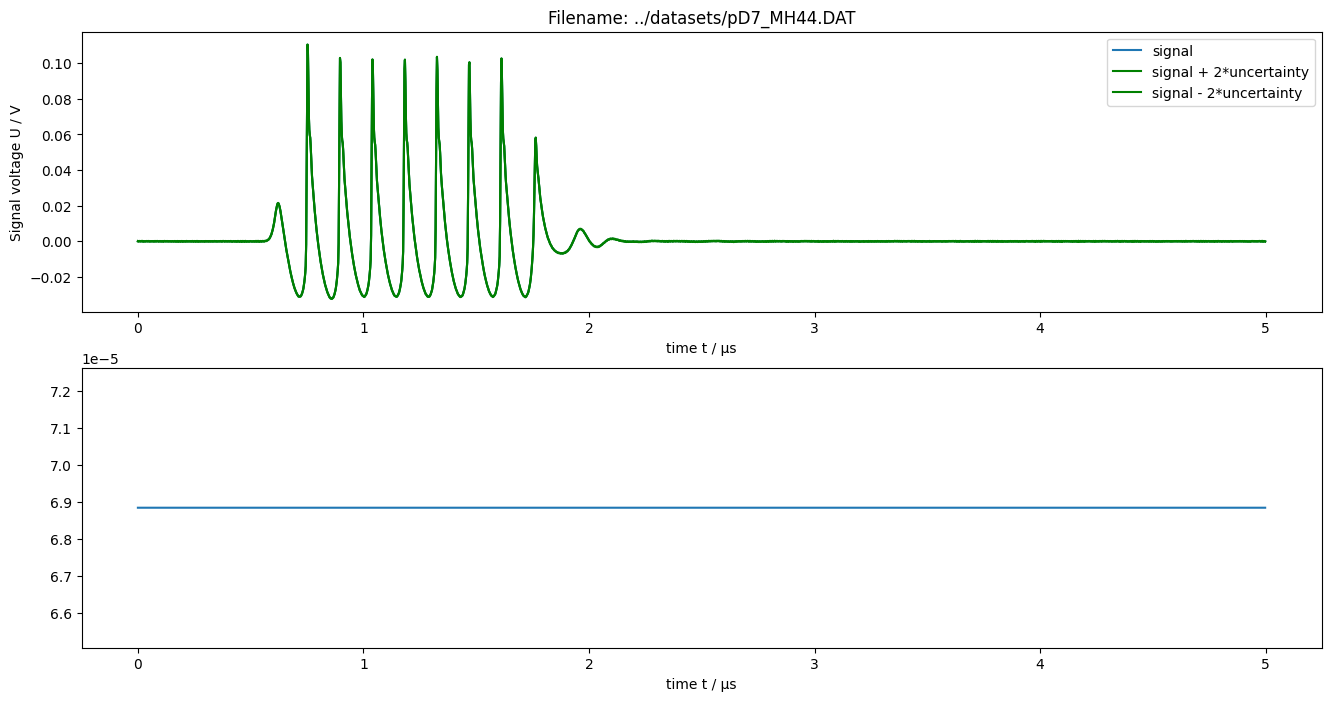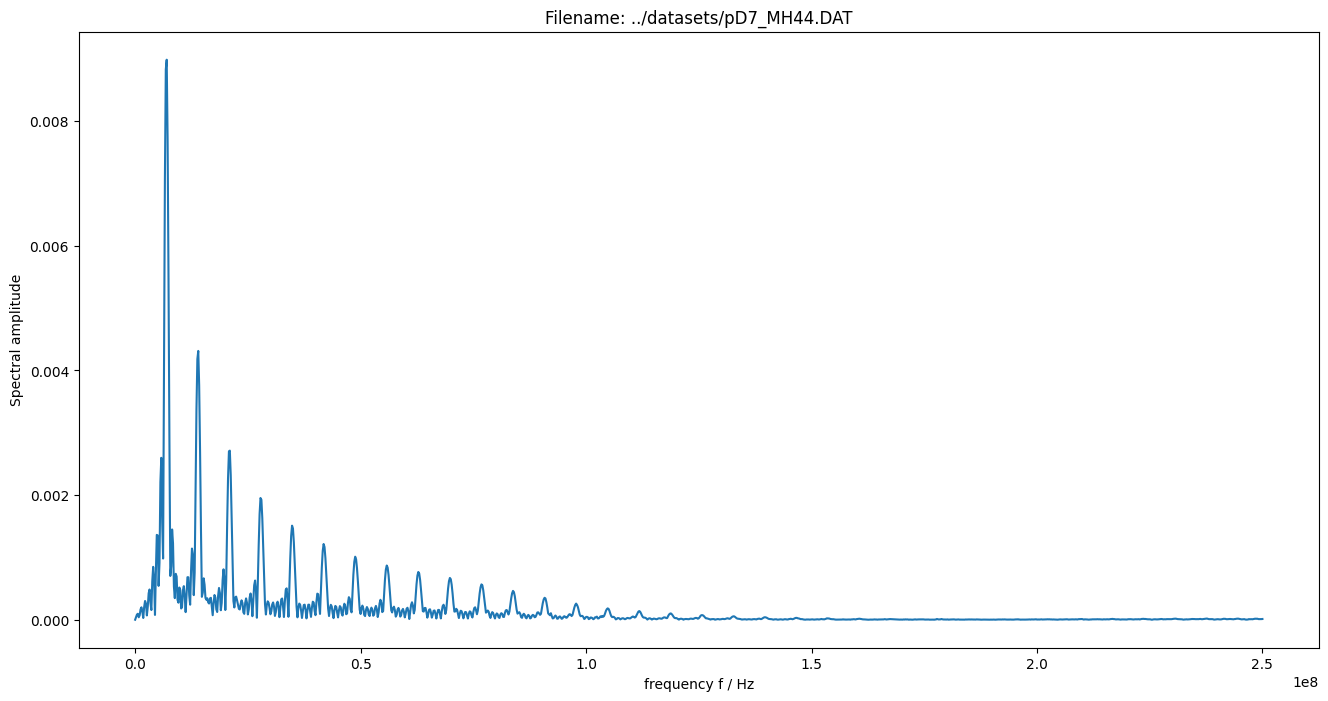# 1. Basic measurement data pre-processing¶

:

%pylab inline

Populating the interactive namespace from numpy and matplotlib

:

from meas_data_preprocessing import *

/home/ludwig10/code/envs/PyDynamic_tutorials-py38/lib/python3.8/site-packages/PyDynamic/identification/fit_filter.py:24: DeprecationWarning: The module *identification* will be combined with the module *deconvolution* and renamed to *model_estimation* in the next major release 2.0.0. From version 1.4.1 on you should only use the new module *model_estimation* instead.
warnings.warn(
/home/ludwig10/code/envs/PyDynamic_tutorials-py38/lib/python3.8/site-packages/PyDynamic/identification/fit_transfer.py:23: DeprecationWarning: The package *identification* will be combined with the package *deconvolution* and renamed to *model_estimation* in the next major release 2.0.0. From version 1.4.1 on you should only use the new package *model_estimation* instead.
warnings.warn(
/home/ludwig10/code/envs/PyDynamic_tutorials-py38/lib/python3.8/site-packages/PyDynamic/uncertainty/interpolation.py:24: PendingDeprecationWarning: The module :mod:PyDynamic.uncertainty.interpolation will be renamed to :mod:PyDynamic.uncertainty.interpolate in the next major release 2.0.0. From version 1.4.3 on you should only use the new module instead.
warnings.warn(


## Read data for a selected measurement scenario¶

:

infos, measurement_data = read_data(meas_scenario = 13)

/home/ludwig10/code/envs/PyDynamic_tutorials-py38/lib/python3.8/site-packages/ipykernel/ipkernel.py:283: DeprecationWarning: should_run_async will not call transform_cell automatically in the future. Please pass the result to transformed_cell argument and any exception that happen during thetransform in preprocessing_exc_tuple in IPython 7.17 and above.
and should_run_async(code)

Checking if file ../datasets/pD7_MH44.DAT is already present or download it from https://raw.githubusercontent.com/Ma-Weber/Tutorial-Deconvolution/master/MeasuredSignals/pD-Mode%207%20MHz/pD7_MH44.DAT otherwise:

file_sizes: 100%|███████████████████████████| 30.5k/30.5k [00:00<00:00, 925kB/s]

file_sizes: 100%|███████████████████████████| 32.7k/32.7k [00:00<00:00, 964kB/s]

file_sizes: 100%|████████████████████████████| 171k/171k [00:00<00:00, 1.88MB/s]
The file ../datasets/pD7_MH44.DAT was read and it contains 2500 data points.
The time increment is 2e-09 s

:

# metadata for chosen measurement scenario
for key in infos.keys():
print("%20s: %s" %(key,infos[key]))

                   i: 13
hydrophonname: GAMPT MH44
measurementtype: Pulse-Doppler-Mode 7 MHz
measurementfile: ../datasets/pD7_MH44.DAT
noisefile: ../datasets/pD7_MH44r.DAT
hydfilename: ../datasets/MW_MH44ReIm.csv

:

# available measurement data
for key in measurement_data.keys():
print("%10s: %s"%(key, type(measurement_data[key])))

      name: <class 'str'>
voltage: <class 'numpy.ndarray'>
time: <class 'numpy.ndarray'>

:

figure(figsize=(18,8))
plot(measurement_data["time"], measurement_data["voltage"])
xlabel("time in s"); ylabel("signal voltage in V")
title(measurement_data["name"]);## Remove DC component¶

:

measurement_data = remove_DC_component(measurement_data)

/home/ludwig10/code/envs/PyDynamic_tutorials-py38/lib/python3.8/site-packages/ipykernel/ipkernel.py:283: DeprecationWarning: should_run_async will not call transform_cell automatically in the future. Please pass the result to transformed_cell argument and any exception that happen during thetransform in preprocessing_exc_tuple in IPython 7.17 and above.
and should_run_async(code)

:

figure(figsize=(18,8))
plot(measurement_data["time"], measurement_data["voltage"])
xlabel("time in s"); ylabel("signal voltage in V")
title(measurement_data["name"]);## Calculate measurement uncertainty from noise data¶

:

measurement_data = uncertainty_from_noisefile(infos, measurement_data, do_plot=False)

The file "../datasets/pD7_MH44r.DAT" was read and it contains 2500 data points

/home/ludwig10/code/envs/PyDynamic_tutorials-py38/lib/python3.8/site-packages/ipykernel/ipkernel.py:283: DeprecationWarning: should_run_async will not call transform_cell automatically in the future. Please pass the result to transformed_cell argument and any exception that happen during thetransform in preprocessing_exc_tuple in IPython 7.17 and above.
and should_run_async(code)

:

# available measurement data
for key in measurement_data.keys():
print("%12s: %s"%(key, type(measurement_data[key])))

        name: <class 'str'>
voltage: <class 'numpy.ndarray'>
time: <class 'numpy.ndarray'>
uncertainty: <class 'numpy.ndarray'>

:

figure(figsize=(16,8))
subplot(211)
plot(measurement_data["time"] / 1e-6, measurement_data["voltage"])
plot(measurement_data["time"] / 1e-6, measurement_data["voltage"] + 2 * measurement_data["uncertainty"], "g")
plot(measurement_data["time"] / 1e-6, measurement_data["voltage"] - 2 * measurement_data["uncertainty"], "g")
legend(["signal", "signal + 2*uncertainty", "signal - 2*uncertainty"])
xlabel("time t / µs")
ylabel("Signal voltage U / V")
title("Filename: {}".format(measurement_data["name"]))

subplot(212)
plot(measurement_data["time"] / 1e-6, measurement_data["uncertainty"])
xlabel("time t / µs");## Calculate spectrum of measured data¶

:

measurement_data = calculate_spectrum(measurement_data, do_plot = False)

/home/ludwig10/code/envs/PyDynamic_tutorials-py38/lib/python3.8/site-packages/ipykernel/ipkernel.py:283: DeprecationWarning: should_run_async will not call transform_cell automatically in the future. Please pass the result to transformed_cell argument and any exception that happen during thetransform in preprocessing_exc_tuple in IPython 7.17 and above.
and should_run_async(code)

:

# available measurement data
for key in measurement_data.keys():
print("%12s: %s"%(key, type(measurement_data[key])))

        name: <class 'str'>
voltage: <class 'numpy.ndarray'>
time: <class 'numpy.ndarray'>
uncertainty: <class 'numpy.ndarray'>
frequency: <class 'numpy.ndarray'>
spectrum: <class 'numpy.ndarray'>
varspec: <class 'numpy.ndarray'>

:

figure(figsize=(16,8))
plot(realpart(measurement_data["frequency"]), amplitude(measurement_data["spectrum"]))
xlabel("frequency f / Hz")
ylabel("Spectral amplitude")
title("Filename: {}".format(measurement_data["name"]));